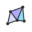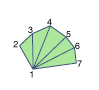...

Chasys Draw IES Help:Triangle List ToolThis tool draws a series of triangles in a polygon fan manner. What you do with it is mostly up to you. Set the starting point and subsequent end points using the left mouse button, then use the right mouse button to end the fan. The points you set take on the current color#1, such that you may choose red, set a point, choose blue, set the second point, and so forth. If you select a point by mistake, you can unselect it by left-clicking while holding shift.

Triangles form the basis of all 3D graphics today, be it your 3D game or 3D modeling application. You can use triangle strips and triangle fans to specify an entire surface without having to provide all three vertices for each of the triangles. For example, only seven vertices are required to define the following triangle fan.The graphics engine uses vertices 1, 2, and 3 to draw the first triangle; vertices 1, 3, and 4 to draw the second triangle; vertices 1, 4, and 5 to draw the third; vertices 1, 5, and 6 to draw the fourth; and so on. Notice that all the triangle share the vertex 1.

Triangles can also be arranged to form a triangle strip.The graphics engine uses vertices 1, 2, and 3 to draw the first triangle; vertices 2, 4, and 3 to draw the second triangle; vertices 3, 4, and 5 to draw the third; vertices 4, 6, and 5 to draw the fourth; and so on. Notice that the vertices of the second and fourth triangles are out of order. This is required to make sure that all of the triangles are drawn in a clockwise orientation. In other words, draw in a zigzag manner, up and down along the line of reference.Working by joining triangles like this, you can create objects such as the one shown above. The pyramid was done using a single triangle fan, and the cuboid was done using four triangle strips.

These are the Tool-box, Palette and Layers windows, respectively. For help on how to use them, consult the User Interface article.